#### 期刊菜单

Analysis of the Relationship between Industrial Waste Discharge and Per Capita GDP in Chengdu—A Metrological Analysis Based on Environmental Kuznets Curve (EKC)
DOI: 10.12677/SD.2020.103043, PDF, HTML, XML, 下载: 441  浏览: 975  科研立项经费支持

Abstract: Based on the environmental Kuznets theory, taking Chengdu as an example, this paper uses Python to estimate the EKC curve of the data. The research results show that the emissions of pollutants in Chengdu from 1997 to 2018 have a correlation with GDP per capita and are in line with the environmental Kuznets curve; among them, the GDP per capita and the amount of industrial wastewater per capita discharge show an inverted “U” Curve; the relationship between industrial exhaust per capita emissions and GDP per capita shows an inverted “N” curve. The relationship between the discharge of industrial solid waste per capita emissions and GDP per capita shows an inverted “U” curve.

1. 引言与文献综述

2. 数据来源及研究方法

2.1. 数据来源Table 1. Statistics of GDP per capita and the three wastes

2.2. 研究方法

2.2.1. 方法概述

2.2.2. 模型介绍

$\left(1-{x}^{2}\right){y}^{″}-x{y}^{\prime }+{n}^{2}y=0$ (1)

${T}_{0}\left(x\right)=1$ (2)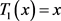(3)

${T}_{n+1}\left(x\right)=2x{T}_{n}\left(x\right)-{T}_{n-1}\left(x\right)$ (4)

x定义域为[−1, 1]。

$Y={b}_{0}+{b}_{1}x$ (5)

$Y={b}_{0}+{b}_{1}x+{b}_{2}{x}^{2}$ (6)

$Y={b}_{0}+{b}_{1}x+{b}_{2}{x}^{2}+{b}_{3}{x}^{3}$ (7)

1) 方程式在 ${b}_{1}={b}_{2}={b}_{3}=0$ 的情况下则表示经济增长不会对环境质量状况产生影响。

2) 方程式在 ${b}_{1}>0,{b}_{2}={b}_{3}=0$ 的情况下表示经济发展与环境状况呈现线性正相关关系，也就是说经济增长会带来环境质量状况的恶化。

3) 方程式在 ${b}_{1}<0,{b}_{2}={b}_{3}=0$ 的情况下，经济发展水平与环境质量状况呈现线性负相关关系，表示经济增长不会引起环境状况的恶化。

4) 在 ${b}_{1}>0,{b}_{2}<0,{b}_{3}=0$ 的情况下，经济发展与环境状况与环境库兹涅茨曲线相吻合，呈现出倒“U”型的曲线关系，也就是说环境质量状况会逐渐恶化而后会得以改善，逐渐转好。

5) 在 ${b}_{1}<0,{b}_{2}>0,{b}_{3}=0$ 的情况下，经济增长与环境质量状况恰恰与环境库兹涅茨曲线相反，呈现出“U”型曲线关系，这意味着随着经济的增长，环境质量状况会变好而后逐渐恶化。

6) 在 ${b}_{1}>0,{b}_{2}<0,{b}_{3}>0$ 的情况下，经济增长与环境呈现“N”型关系，表示环境质量状况随经济发展先变好再恶化，当经济持续增长时环境质量状况会再次转好。

7) 在 ${b}_{1}<0,{b}_{2}>0,{b}_{3}<0$ 的情况下，经济增长与环境呈现倒“N”型关系，意味着随着经济的增长，环境质量状况会逐渐恶化而后会得以改善，最后再次呈现下降趋势。

8) 当 ${b}_{1}={b}_{2}={b}_{3}=0$ 时特殊状况出现，表示环境污染与经济水平无相关关系 。

3. 成都市经济发展指标与环境污染方程拟合结果分析

3.1. 成都市人均GDP的方程拟合Table 2. Chebyshev polynomial curve equation and Test of fitness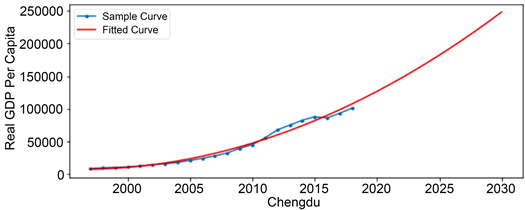Figure 1. Chengdu GDP per capitaTable 3. Forecast Chengdu’s GDP per capita from 2019 to 2030

3.2. 工业废水人均排放量的方程拟合Table 4. Fitting results of industrial wastewater per capita discharge and per capita GDP equation in Chengdu

$Y=-2.551\text{e}\text{ }-\text{ }10{X}^{3}+3.043\text{e}\text{ }-\text{ }05{X}^{2}-0.9278x+3.619\text{e}\text{ }+\text{ }04$ (8)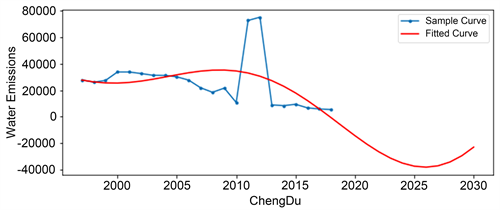Figure 2. Quadratic curve model of industrial wastewater per capita discharge and GDP per capita in Chengdu

3.3. 工业废气人均排放量的方程拟合Table 5. Fitting results of industrial exhaust per capita emissions and per capita GDP equation in Chengdu

$Y=8.699\text{e}\text{ }-\text{ }11{x}^{3}-1.975\text{e}\text{ }-\text{ }05{x}^{2}+1.345x-5082$ (9)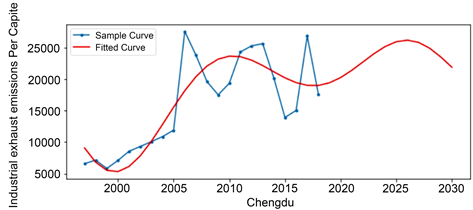Figure 3. The cubic curve model of industrial exhaust per capita emissions and GDP per capita in ChengduTable 6. Chengdu City Industrial exhaust per capita Emissions and Per Capita GDP Forecast

3.4. 工业固体废弃物人均排放总量的方程拟合Table 7. Fitting results of industrial solid waste per capita emissions and per capita GDP equation in Chengdu

$Y=1.867\text{e}\text{ }-\text{ }12{x}^{3}-4.503\text{e}\text{ }-\text{ }07{x}^{2}+0.0276x+48.6400$ (10)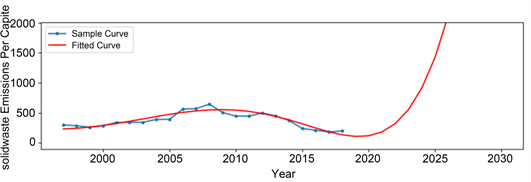Figure 4. Cubic model of industrial solid waste per capita emissions and GDP per capita in ChengduTable 8. Forecast of Chengdu’s industrial solid waste per capita emissions in 2019 to 2030

4. 结论与建议

  穆红莉. 北京市环境库兹涅茨曲线特征分析[J]. 生态 经济(学术版), 2008(1): 366-368, 380.  刘璨, 吕金芝. 中国森林资源环境库兹涅茨曲线问题研究[J]. 制度经济学研究, 2010(2): 138-161.  成舸, 岳贤平. 基于环境库兹涅茨曲线的经济增长与碳排放相关性研究: 以江苏为例[J]. 商场现代化, 2011(5): 92-94.  魏雪梅. 基于库兹涅茨曲线的福州市经济发展与环境污染关系研究[J]. 绿色科技, 2019(24): 143-147.  王懿. 安徽省环境库兹涅茨曲线现状分析[J]. 蚌埠学院学报, 2020(2): 49.  朱冉. 成都市在产业转移下的经济增长和污染:环境库兹涅茨曲线的启示[C]//中国地理学会经济地理专业委员会. 2017年中国地理学会经济地理专业委员会学术年会论文摘要集. 中国地理学会经济地理专业委员会: 中国地理学会, 2017: 96.  王先传, 江岩, 赵佳, 张岩, 等. 基于切比雪夫多项式的函数插值逼近[J]. 阜阳师范学院学报(自然科学版), 2017(4): 3-11.  王锋君. 实施“143”行动计划, 推动成都生态环境保护[J]. 先锋, 2017(2): 43-44.